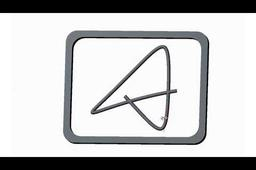creo parametric | Wall art decor design using Curve methodll learn create curve using equation

EQUATIONS

Cartesian Coordinates: x, y, & z

The z variable is not necessary, but when used will give the curve that extra dimension.

If in doubt, try z = t*10.

Rhodonea
Cartesian coordinates
theta = t * 360 * 4
x = 25 + (10-6) * cos (theta) +10 * cos ((10/6-1) * theta)
y = 25 + (10-6) * sin (theta) - 6 * sin ((10/6-1) * theta)